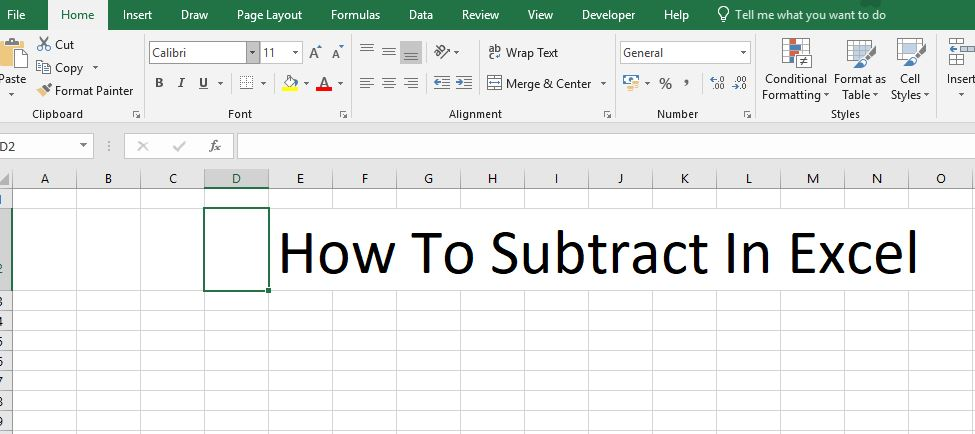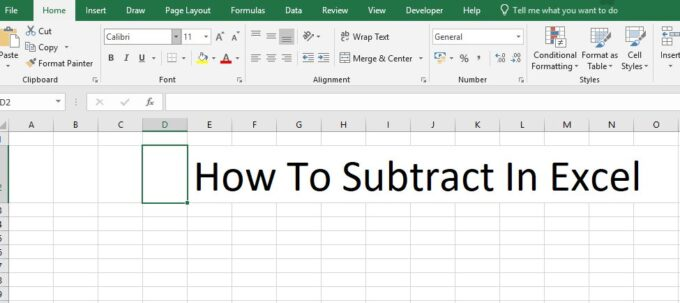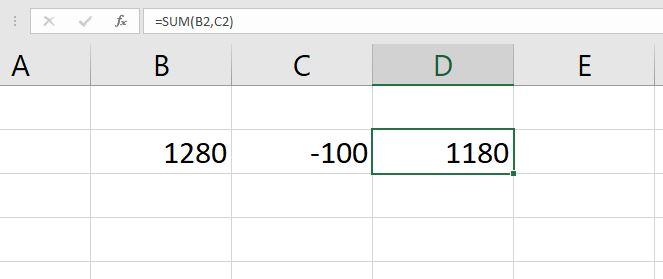# How to Subtract in Excel

Learn how to subtract in Excel with this valuable how-to guide. This article will walk you through each step of the process from start to finish.

Excel is a powerful program that makes organizing numbers and data easy for anyone. But, learning how to perform even simple functions can be a bit tricky when first starting out. Excel can perform many different functions and one of the most basic is subtraction. Below you will find a complete guide on how to subtract in Excel.## Different Types of Excel Subtraction

As mentioned above, Excel can subtract numbers in a single cell or within a range of cells. Both operations are simple to perform and only a little different from one another.

Below, we will talk about the different ways to subtract in Excel and give you some examples.

To get the most out of the information below, keep these terms in mind while reading:

• Worksheet: an electronic document made up of rows and columns that can contain data
• Cell: the intersection of a row and a column on a worksheet
• Formula: the instructions entered into a cell to produce a specific result
• Function: a built-in formula used in Excel

## Subtracting with Simple Numbers

For simple math problems, you can use a single cell to calculate subtraction problems. As an example, we’ll use the problem 5 – 4 = 1. This problem is simple, but you can apply the same concept to larger numbers and more complex data.

To begin, use your cursor to select an empty cell on the worksheet. Once you select the cell, begin to type your formula.

In Excel, all formulas start with an equal sign (=). After you’ve typed the equal sign, type the numbers you’re subtracting separated by the minus sign (-). In this case, your cell would contain the characters “=5-4.”

Once you have entered the numbers you’d like to subtract, hit the “Enter” key.

Hitting the “Enter” key tells Excel that you are ready to execute your formula. The data in the cell will transform from the formula you entered to the solution of that formula. The example cell would now read “1” instead of “=5-4.”

## Subtraction Using Two or More Unique Cells

In Excel, every cell has a “name” made by combining its column letter with its row number.

This is the cell reference. For example, the cell created where column A intersects row 1 is cell A1. You can use cell references in formulas to execute various operations including subtraction.

Like before, this type of subtraction begins by selecting an empty cell.

Follow the same steps, but, instead of entering numbers, enter specific cell references. For example, if you’d like to subtract the quantity in cell A1 from the quantity in cell B1, your formula would read “=B1-A1.”

Instead of typing in a cell, you can also type formulas into the formula bar found at the top of the worksheet. You can also select cells with your cursor after starting your formula instead of typing them out.

## How to Subtract Using the SUM Function

As mentioned earlier, functions are Excel’s built-in formulas. A variety of functions are available in Excel. When subtracting in excel, the SUM function is most useful.

Although addition and subtraction are often thought of as opposites they are, in fact, one and the same.

While we may not think about it, subtracting a number is the same as adding a negative number. Excel does not have a SUBTRACTION function but instead relies upon its built-in SUM function.

Excel’s SUM function can use individual numbers, cell references, or a range of cells.

To subtract numbers using the SUM function, make the number you want to subtract a negative value. For example, we’ll say that cell A1 contains the number 5 and cell A2 contains the number 3. You can use the SUM function in an empty cell to subtract 3 from 5.

First, make the number you want to subtract negative by adding a minus sign (-) to it. In this example, we are subtracting 3 from 5 so we will add the minus sign (-) to the 3 in cell A2 making it -3.

To use the SUM function, enter an equal sign into an empty cell followed by the word SUM.

The equal sign tells Excel that you will be using a formula. The word SUM specifies the function you want to use. In parentheses after the word SUM, press enter for the numbers, cell references, or range of cells in Excel you want to sum.### For the example given above, your SUM function would look like one of the following:

• If you used individual numbers, “=SUM(5,-3)”
• For using cell references, “=SUM(A1, A2)”
• If you selected a range of cells, “=SUM(A1: A2)”

In Excel, you can also use the AutoSUM wizard by clicking on the “Formulas” tab and then choosing AutoSUM. Always switch the values you are subtracting to negative when using the SUM function.

## How To Subtract In Excel: Final Review

As you can see, there are several different methods for how to subtract in Excel. Depending upon the type of data you are dealing with, some of these methods will work better than others. You can use each method to subtract numbers both large and small and organize large amounts of data. Learning how to subtract in Excel is a quick and simple process that anyone can master.

With a small amount of patience, you can apply these concepts to any worksheet you come across. Subtraction may seem like an insignificant skill to gain, but it is a step towards harnessing the full power of Excel. Do you want to know more about Excel’s Intermediate features? If yes, then click here.This site uses Akismet to reduce spam. Learn how your comment data is processed.